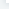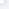# B4A这里是一些内置的关键字

IsInitialized As Boolean
Message As String [只读]

IsInitialized As Boolean
Message As String [只读]
Keywords

Abs (Number As Double) As Double
ACos (Value As Double) As DoubleACosD (Value As Double) As Double
Array
Asc (Char As Char) As Int
ASin (Value As Double) As Double
ASinD (Value As Double) As Double
ATan (Value As Double) As Double
ATanD (Value As Double) As Double
BytesToString (Data() As Byte, StartOffset As Int, Length As Int, CharSet As String)
As String
Catch
cE As Double
CharsToString (Chars() As Char, StartOffset As Int, Length As Int) As String
Chr (UnicodeValue As Int) As Char
Continue
Cos (Radians As Double) As Double
CosD (Degrees As Double) As Double
cPI As Double
CRLF As String
Density As Float
Dim
DipToCurrent (Length As Int) As Int
DoEvents
Exit
ExitApplication
False As Boolean
File As File
Floor (Number As Double) As Double
For
GetDeviceLayoutValues As LayoutValues
GetType (object As Object) As String
If
InputList (Items As List, Title As String, CheckedItem As Int) As Int
InputMultiList (Items As List, Title As String) As List
Is
Boolean
IsNumber (Text As String) As Boolean
LastException As Exception
LoadBitmap (Dir As String, FileName As String) As Bitmap
LoadBitmapSample (Dir As String, FileName As String, MaxWidth As Int,MaxHeight As Int) As Bitmap
Log (Message As String)
Logarithm (Number As Double, Base As Double) As Double
Max (Number1 As Double, Number2 As Double) As Double
Min (Number1 As Double, Number2 As Double) As Double
Msgbox (Message As String, Title As String)
Msgbox2 (Message As String, Title As String, Positive As String, Cancel As String,Negative As String, Icon As android.graphics.Bitmap) As Int
Not (Value As Boolean) As Boolean
Null As Object
NumberFormat (Number As Double, MinimumIntegers As Int, MaximumFractions As Int) As String
NumberFormat2 (Number As Double, MinimumIntegers As Int, MaximumFractions As Int, MinimumFractions As Int, GroupingUsed As Boolean) As String
PerXToCurrent (Percentage As Int) As Int
PerYToCurrent (Percentage As Int) As Int
Power (Base As Double, Exponent As Double) As Double
ProgressDialogHide
ProgressDialogShow (Text As String)
ProgressDialogShow2 (Text As String, Cancelable As Boolean)
QUOTE As String
Regex As Regex
Return
Rnd (Min As Int, Max As Int) As Int
Round (Number As Double) As Long
Round2 (Number As Double, DecimalPlaces As Int) As Double
Sender As Object
Sin (Radians As Double) As Double
SinD (Degrees As Double) As Double
Sqrt (Value As Double) As Double
StartActivity (Activity As Object)
Sub
TAB As String
Tan (Radians As Double) As Double
TanD (Degrees As Double) As Double
ToastMessageShow (Message As String, LongDuration As Boolean)
True As Boolean
Try
Type
Until

B4A这里是一些内置的关键字

## 频道栏目导航